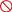# Machine Learning Python: Regression Modeling - Price: Rs. 750Contact
Ben Cryer
Phone

[not verified]City
Bangalore
Zip
560017 (map)
Created
October 9, 2019
Updated
October 9, 2019
Expires
October 8, 2020
Viewed
17
The course "Machine Learning Basics: Building Regression Model in Python" teaches you all the steps of creating a Linear Regression model, which is the most popular Machine Learning model, to solve business problems.

In this course students will learn the following:

How to predict future outcomes basis past data by implementing Simplest Machine Learning algorithm
How to do preliminary analysis of data using Univariate and Bivariate analysis before running Linear regression
Understand how to interpret the result of Linear Regression model and translate them into actionable insight
Understanding of basics of statistics and concepts of Machine Learning
Learn advanced variations of OLS method of Linear Regression
Linear Regression technique of Machine Learning using Scikit Learn and Statsmodel libraries of Python
This course is suitable for anyone curious about machine learning or professionals beginning their data journey.

Basic knowledge
Students will need to install Python and Anaconda software but we have a separate lecture to help you install the same
What will you learn
In this course students will learn the following:

How to predict future outcomes basis past data by implementing Simplest Machine Learning algorithm
How to do preliminary analysis of data using Univariate and Bivariate analysis before running Linear regression
Understand how to interpret the result of Linear Regression model and translate them into actionable insight
Understanding of basics of statistics and concepts of Machine Learning
Learn advanced variations of OLS method of Linear Regression
Linear Regression technique of Machine Learning using Scikit Learn and Statsmodel libraries of Python

https://www.simpliv.com/machinelearning/machine-learning-basics-regression-modeling-in-python
It & Software System Courses Online | Simpliv (Bangalore,) Training & Education Services
Machine Learning With Aws (Bangalore,) Training & Education Services
Learn By Example: Statistics And Data Science In R (Bangalore,) Training & Education Services